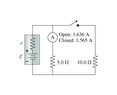# How to solve for EMF and internal resistance in a circuit?

np115
Homework Statement:
What is the emf of the battery in the figure(Figure 1)?
What is the internal resistance of the battery in the figure?
Relevant Equations:
V=iR
V=E-ir
I know the answers are 9 V and .5 ohms but I have no idea how to get there. Originally I solved for Delta V in the open circuit through (1.636)(5) which gave me 8.18V. Then, I solved for Delta V in the closed circuit through (1.565)(1/((1/5)+(1/10)) which gave me 5.51 V. I used these voltages to set up the equations using V=E-ir. *this is something that really confuses me! is this equation referring to the total resistance in the circuit or just the internal resistance in the battery? If it is total, how would I put this into the equation?* Using that equation I got 8.18 V = E -(1.636 (r+5)) and 5.21 = E - (1.565(r+3.33)). Then, I used elimination to solve but got really confused because things do not add up. I have a quiz tomorrow over this and I have NO IDEA WHAT I"M DOING! Really appreciate some help!

#### Attachments

•EMopen:closed.jpeg
6 KB · Views: 41

Staff Emeritus
2022 Award
I know the answers are 9 V and .5 ohms but I have no idea how to get there. Originally I solved for Delta V in the open circuit through (1.636)(5) which gave me 8.18V.
How did you do this? You don't know R of the open circuit because you don't know r of the battery. So it's V=IR=1.565*(5+r), which can't yet be solved.
Similar situation for the closed circuit.

Try setting up your two voltage equations and making them equal to each other. That gets rid of V, leaving you with two equations and 1 unknown variable, which is solvable.

np115
wait why would it be 1.565(r+5)? that's the current value for the open circuit. So shouldn't it be 1.636(r+5)? and when I solved that by setting both equations equal to each other, i got r=-41.8 which doesn't make any sense. And when I tried solving it with your equations, I got r=33.45.

Homework Helper
2022 Award
Could you solve for the two currents if instead you were told the battery V and r and not the two currents?

np115
I'm sure you could since the change in voltage for both circuits would still be the same so if you put both equations equal to each other, you could solve for i.

Staff Emeritus
2022 Award
ait why would it be 1.565(r+5)? that's the current value for the open circuit. So shouldn't it be 1.636(r+5)?
My apologies, I mixed up the values and for some reason thought the ammeter was located on the top wire before it branched off to the 5 ohm resistor. Getting only 1 current value for the 2-branch circuit makes things much more difficult. It's been a while since I did basic circuits. Let me give it some thought and get back to you.

Staff Emeritus
2022 Award
Ok, here we go.
First, find all the info you can for your first circuit (when switch is open). You know the current, so you know the voltage across the resistor.

Now, do the same thing for the circuit when the switch is closed. Notice that when the switch is closed, you have ##R_5## and ##R_{10}## in parallel, and both are in series with ##R_1##, the battery's resistor. Remember that parallel resistors have something in common (hint: it starts with a V). We want the current through both branches (we have one already).

Once you have the current through both branches, you know the current through the battery for both circuits (they gave us the current for the first one). Use these, try some KVL and Ohm's law, and if you need more help let me know.

Last edited:
np115
I got it, thank you guys so much for your help!

•Drakkith, berkeman and hutchphd
Staff Emeritus